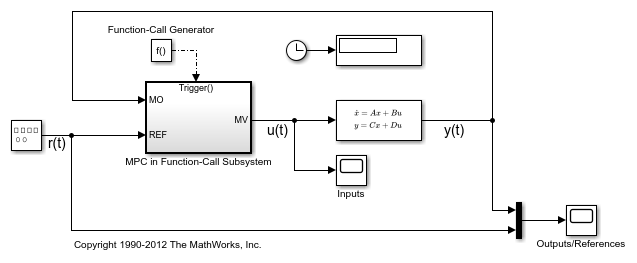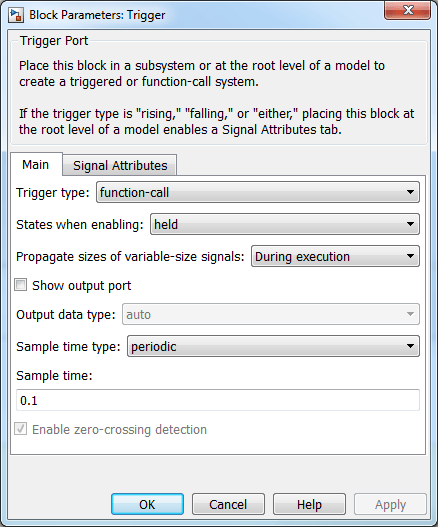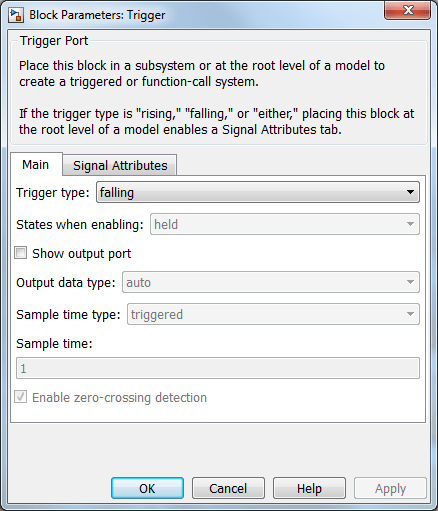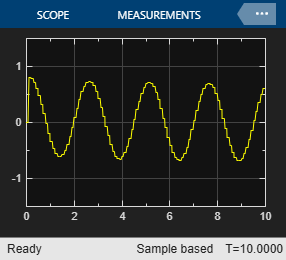# Using MPC Controller Block Inside Function-Call and Triggered Subsystems

This example shows how to configure and simulate MPC Controller blocks placed inside Function-Call and Triggered subsystems.

### Define Plant Model and MPC Controller

Define a plant.

```plant = ss(tf([3 1],[1 0.6 1])); ```

Define the MPC controller for the plant.

```Ts = 0.1; % Sampling time p = 10; % Prediction horizon m = 2; % Control horizon Weights = struct('MV',0,'MVRate',0.01,'OV',1); % Weights MV = struct('Min',-Inf,'Max',Inf,'RateMin',-100,'RateMax',100); % Input constraints OV = struct('Min',-2,'Max',2); % Output constraints mpcobj = mpc(plant,Ts,p,m,Weights,MV,OV); ```

### Configure and Simulate MPC Controller Block Inside Function-Call Subsystem

Function-Call subsystem is invoked directly by another block during simulation. If you invoke the Function-Call subsystem periodically with the same sample time specified in the MPC controller object then you get exactly the same behavior as an MPC controller block placed directly in a feedback loop with your plant, (without being in a subsystem) and that does not inherit its same sample time. If you must use a different sample time, then you should:

• make sure that the manipulated variable rate (which depends on the last value of the manipulated variable) is handled correctly in the controller weights and constraints,

• enable custom estimation instead of using built-in estimation, as the built-in estimator uses the sample time in the MPC object to provide a state estimate for the MPC optimization problem.

Open the model.

```mdl1 = 'mpc_rtwdemo_functioncall'; open_system(mdl1) ```The reference signal is a sine wave with amplitude `1` and frequency of `0.4` Hz. The MPC Controller block is inside the MPC in Triggered Subsystem block.

```open_system([mdl1 '/MPC in Function-Call Subsystem']) ```Configure the controller to use an inherited sample time. To do so, select the Inherit sample time property of the MPC Controller block.

Invoke the Function-Call subsystem periodically with the correct sample time.

For this example, since the controller has a sample time of 0.1 seconds, configure the trigger block inside the Function-Call subsystem to use the same sample time.For this example, use the Function-Call Generator block to execute the Function-Call subsystem at the sample rate as 0.1 seconds.Simulate the model.

```close_system([mdl1 '/MPC in Function-Call Subsystem/MPC Controller']) open_system([mdl1 '/Inputs']) open_system([mdl1 '/Outputs//References']) sim(mdl1) ```
```-->Converting model to discrete time. -->Assuming output disturbance added to measured output #1 is integrated white noise. -->"Model.Noise" is empty. Assuming white noise on each measured output. ```The controller effort and the plant output are saved into base workspace (by the `To Workspace` blocks in the function call subsystem) as the variables `u_fc` and `y_fc`, respectively.

```bdclose(mdl1) ```

### Configure and Simulate MPC Controller Block Inside Triggered Subsystem

Triggered subsystem executes each time a trigger event occurs. The same considerations on inheriting the sample time made earlier for the Function Call subsystem apply.

Open the model.

```mdl2 = 'mpc_rtwdemo_triggered'; open_system(mdl2) ```The MPC Controller block is in the MPC in Triggered Subsystem block.

```open_system([mdl2 '/MPC in Triggered Subsystem']); ```Configure the MPC block to use an inherited sample time, as you did for the function-call subsystem model.

Execute the Triggered subsystem periodically with the correct sample time.

For this example, configure the Trigger block inside the triggered subsystem to use a `falling` trigger type.For this example, use the Pulse Generator block to provide a periodic triggering signal at the sample rate as 0.1 seconds.Simulate the model.

```close_system([mdl2 '/MPC in Triggered Subsystem/MPC Controller']) open_system([mdl2 '/Inputs']) open_system([mdl2 '/Outputs//References']) sim(mdl2) ```The controller effort and the plant output are saved into base workspace (by the `To Workspace` blocks in the triggered subsystem) as the variables `u_tr` and `y_tr`, respectively.

```bdclose(mdl2) ```

### Compare Responses

Compare the simulation results from the Function-Call subsystem and the Triggered subsystem with the result generated by an MPC Controller block that is not placed inside a subsystem and does not inherit sample time.

```mdl = 'mpc_rtwdemo'; open_system(mdl) sim(mdl) ```Compare the responses of the manipulated variable.

```figure plot(t,u,'b-',t,u_fc,'ro',t(1:end-1),u_tr,'k.') title('Manipulated Variable') legend('No Subsystem','Function-Call','Triggered') ```Compare the responses the plant output.

```figure plot(t,y,'b-',t,y_fc,'ro',t(1:end-1),y_tr,'k.') title('Plant Output') legend('No Subsystem','Function-Call','Triggered') ```The results of all three models are numerically equal.

```bdclose(mdl) ```